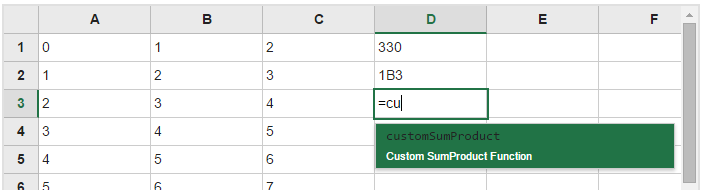The FlexSheet control has a built-in calculation engine that supports over 80 of the functions supported by Excel. (See complete list.) Although the functions provided should cover the vast majority of use scenarios, in some cases users may need additional functions. FlexSheet provides two methods that allow developers to add their own custom functions: addCustomFunction and unknownFunction.

The addCustomFunction method adds a custom function to the list of built-in functions. The method takes the following parameters:

``````
name: string, // name of the new function
function: Function, // new function implementation
description: string, // description of the new function
minParamCount: number, // minimum parameter count for the new function
maxParamCount: number) // maximum parameter count for the new function
``````

For example, the code below adds a custom function called “dec2hex” that converts a decimal number to hexadecimal notation:

`````` flexSheet.addCustomFunction(
'dec2hex',
function(number) {
if (!wijmo.isNumber(number)) {
throw 'Parameter should be a number!'
}
return number.toString(16);
},
'Converts a decimal number to hexadecimal',
1, 1);
``````

The new function will be available for use in any formulas. Additionally, its name and description will appear in the auto-complete list that appears as users type formulas into FlexSheet cells:End users can see your custom function in the formula list (autocomplete dropdown). Try customSumProduct fiddle >>

### unknownFunction Method

The addCustomFunction method is usually the best way to add custom functions to the FlexSheet calculation engine. However, there are scenarios where the function names are variable or unknown ahead of time. Imagine, for example, named ranges or value dictionaries. In these situations, you can use the unknownFunction event to look up the value of a function dynamically. When the FlexSheet detects an unknown function name, it raises the unknownFunction event and provides parameters that contain the function name and parameters. The event handler may then calculate the result and return the value. For example, the code below uses the unknownFunction event to provide a “foo” function that adds all the parameters passed in as arguments:

``````flexSheet.unknownFunction.addHandler(function(sender, e) {
var result = '';
if (e.funcName === 'foo'){
if (e.params) {
for (var i = 0; i < e.params.length; i++) {
result += e.params[i];
}
}
e.value = result;
}
});

``````